# 1 Introduction

If many genes are perturbed in a population of cells, this can lead to diseases like cancer. The perturbations can happen in different ways, e.g. via mutations, copy number abberations or methylation. However, not all perturbations are observed in all samples.

Nested Effects Model-based perturbation inference (NEM$$\pi$$) uses observed perturbation profiles and gene expression data to infer unobserved perturbations and augment observed ones. The causal network of the perturbed genes (P-genes) is modelled as an adjacency matrix $$\phi$$ and the genes with observed gene expression (E-genes) are modelled with the attachment $$\theta$$ with $$\theta_{ij}=1$$, if E-gene $$j$$ is attached to S-gene $$i$$. If E-gene $$j$$ is attached to P-gene $$i$$, $$j$$ shows an effect for a perturbation of P-gene $$i$$. Hence, $$\phi\theta$$ predicts gene expression profiles, which can be compared to the real data. NEM$$\pi$$ iteratively infers a network $$\phi$$ based on gene expression profiles and a perturbation profile, and the perturbation profile based on a network $$\phi$$.

Use devtools to install the latest version from github or use the BiocManahger to install the package from bioconductor.

if (!requireNamespace("BiocManager", quietly = TRUE)) install.packages("BiocManager")
BiocManager::install("nempi")

Load the package with the library function.

library(nempi)

# 3 Small example

We look at a small example for which we first simulate data and then infer the unobserved perturbations. We draw a random network for the perturbed genes (P-genes). Then we simulate gene expression for each sample given the subset of P-genes that has been perturbed in the sample. E.g., if P-gene A is upstream of P-gene B and P-gene A has been perturbed, all E-genes attached to B also show an effect.

## 3.1 Data simulation

library(mnem)
seed <- 8675309
Pgenes <- 10
Egenes <- 5
samples <- 100
edgeprob <- 0.5
uninform <- floor((Pgenes * Egenes) * 0.1)
Nems <- mw <- 1
noise <- 1
multi <- c(0.2, 0.1)
set.seed(seed)
simmini <- simData(Sgenes = Pgenes, Egenes = Egenes, Nems = Nems, mw = mw, nCells = samples,
uninform = uninform, multi = multi, badCells = floor(samples * 0.1), edgeprob = edgeprob)
data <- simmini$data ones <- which(data == 1) zeros <- which(data == 0) data[ones] <- rnorm(length(ones), 1, noise) data[zeros] <- rnorm(length(zeros), -1, noise) epiNEM::HeatmapOP(data, col = "RdBu", cexRow = 0.75, cexCol = 0.75, bordercol = "transparent", xrot = 0, dendrogram = "both")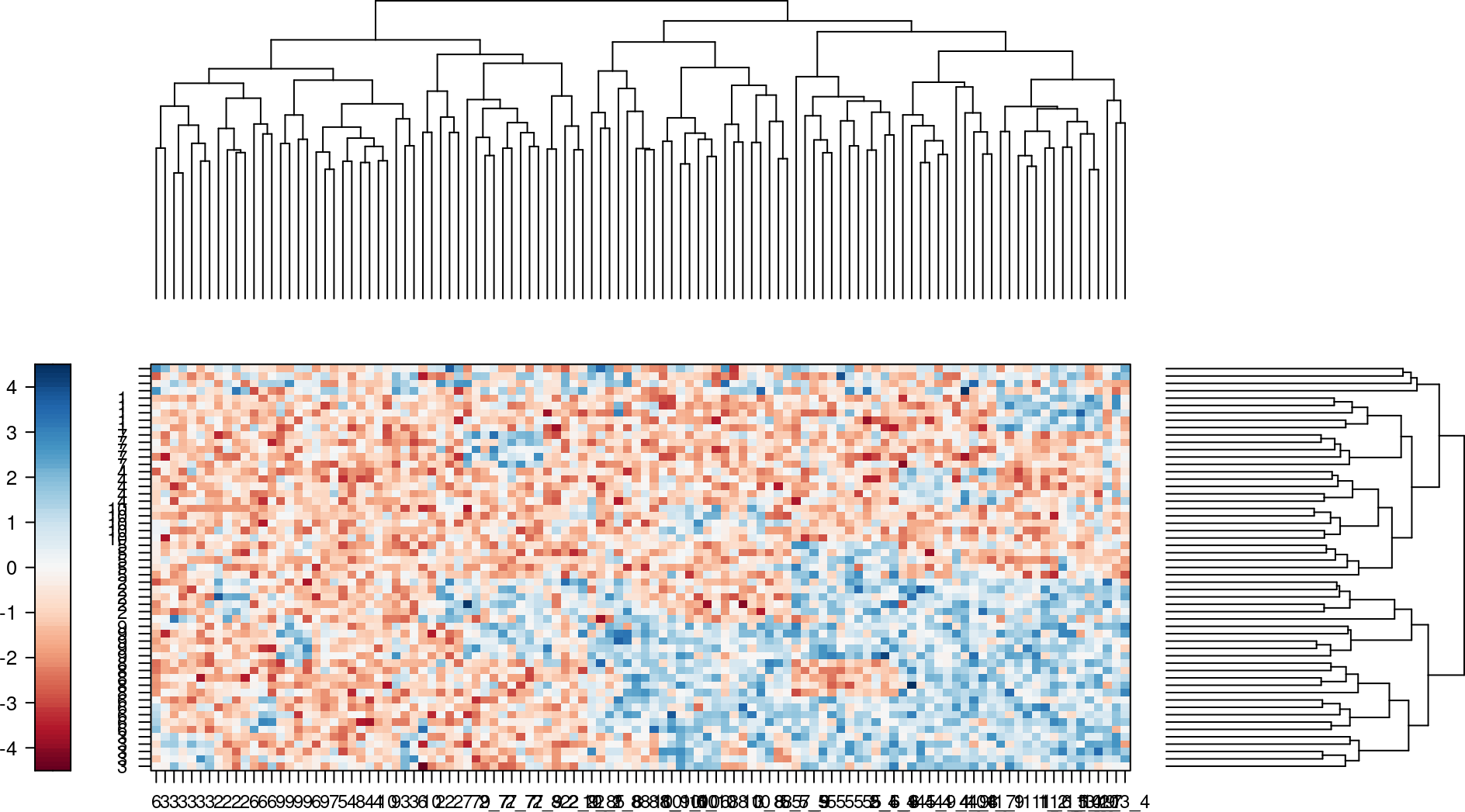Figure 1: Heatmap of the simulated log odds Effects are blue and no effects are red. Rows denote the observed E-genes and columns the samples annoted by P-genes. Each P-gene has been perturbed in many cells. The E-genes are annotated as how they are attached in the ground truth. E.g. E-genes named ‘1’ are attached to S-gene ‘1’ in the ground truth. The typical data input for NEM$$\pi$$ consists of a data matrix with samples as columns and E-genes as rows. The columns are either labeled by their perturbed gene(s) or unlabeled (default: ""). After the data simulation all samples are labeled. We unlabel $$50\%$$ of the sample and pretend we do not know, which P-gene has been perturbed. lost <- sample(1:ncol(data), floor(ncol(data) * 0.5)) colnames(data)[lost] <- "" ## 3.2 Perturbation inference We use NEM$$\pi$$ and other methods to infer the perturbations. We use the area under the precision-recall curve as the two measures of accuracy. We also plot the NEM$$\pi$$ result. res <- nempi(data) fit <- pifit(res, simmini, data) print(fit$auc)
##  0.7788576
ressvm <- classpi(data)
fit <- pifit(ressvm, simmini, data, propagate = FALSE)
print(fit$auc) ##  0.7090377 resnn <- classpi(data, method = "nnet") fit <- pifit(resnn, simmini, data, propagate = FALSE) print(fit$auc)
##  0.6273228
resrf <- classpi(data, method = "randomForest")
fit <- pifit(resrf, simmini, data, propagate = FALSE)
print(fit$auc) ##  0.6987766 col <- rgb(seq(0, 1, length.out = 10), seq(1, 0, length.out = 10), seq(1, 0, length.out = 10)) plot(res, heatlist = list(col = "RdBu"), barlist = list(col = col))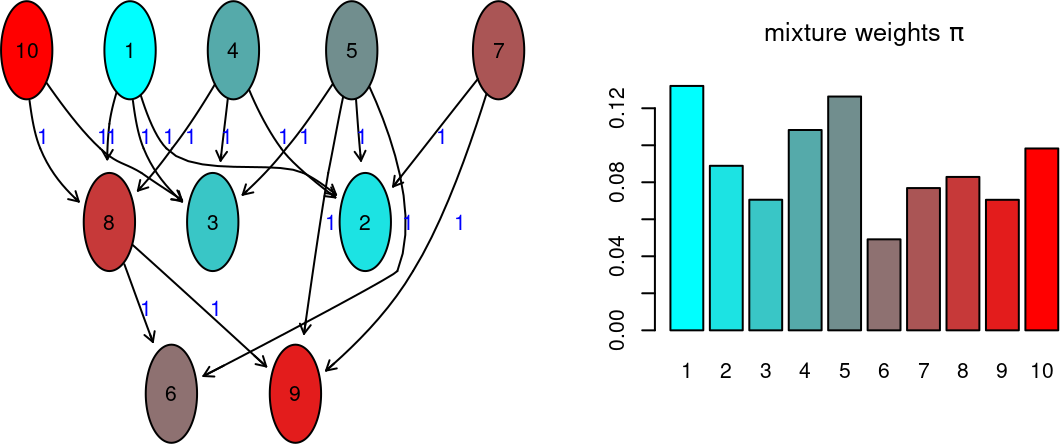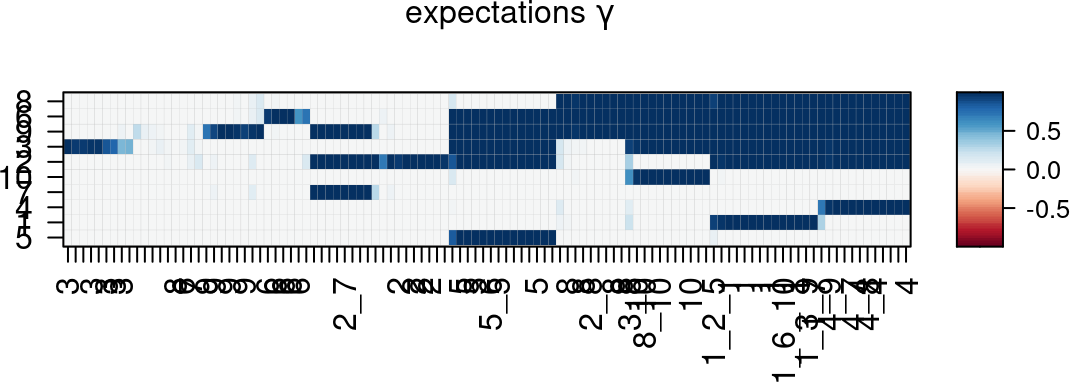Compared to support vector machines (svm), neural nets (nnet) and random forest (rf) class prediction, NEM$$\pi$$ achieves a higher accuracy. Note that NEM$$\pi$$ is in general more powerful, if the P-genes are connected in a denser network. The other methods perform equally well for sparse or even disconnected network $$\phi$$. However, they usually profit from combinatorial perturbations. ## 3.3 Prior matrix Alternatively, we can also provide NEM$$\pi$$ with a probabilistic perturbation matrix $$\Gamma$$ as a prior. In the rows are the potentially perturbed genes and in the columns are the samples. The entries are between $$0$$ and $$1$$, with the sum of all entries of a sample summing to $$1$$. Gamma <- matrix(0, Pgenes, ncol(data)) rownames(Gamma) <- seq_len(Pgenes) colnames(Gamma) <- colnames(data) for (i in seq_len(Pgenes)) { Gamma[i, grep(paste0("^", i, "_|_", i, "$|_", i, "_|^", i, "$"), colnames(data))] <- 1 } Gamma <- apply(Gamma, 2, function(x) return(x/sum(x))) Gamma[is.na(Gamma)] <- 0 epiNEM::HeatmapOP(Gamma, col = "RdBu", cexRow = 0.75, cexCol = 0.75, bordercol = "transparent", xrot = 0, dendrogram = "both")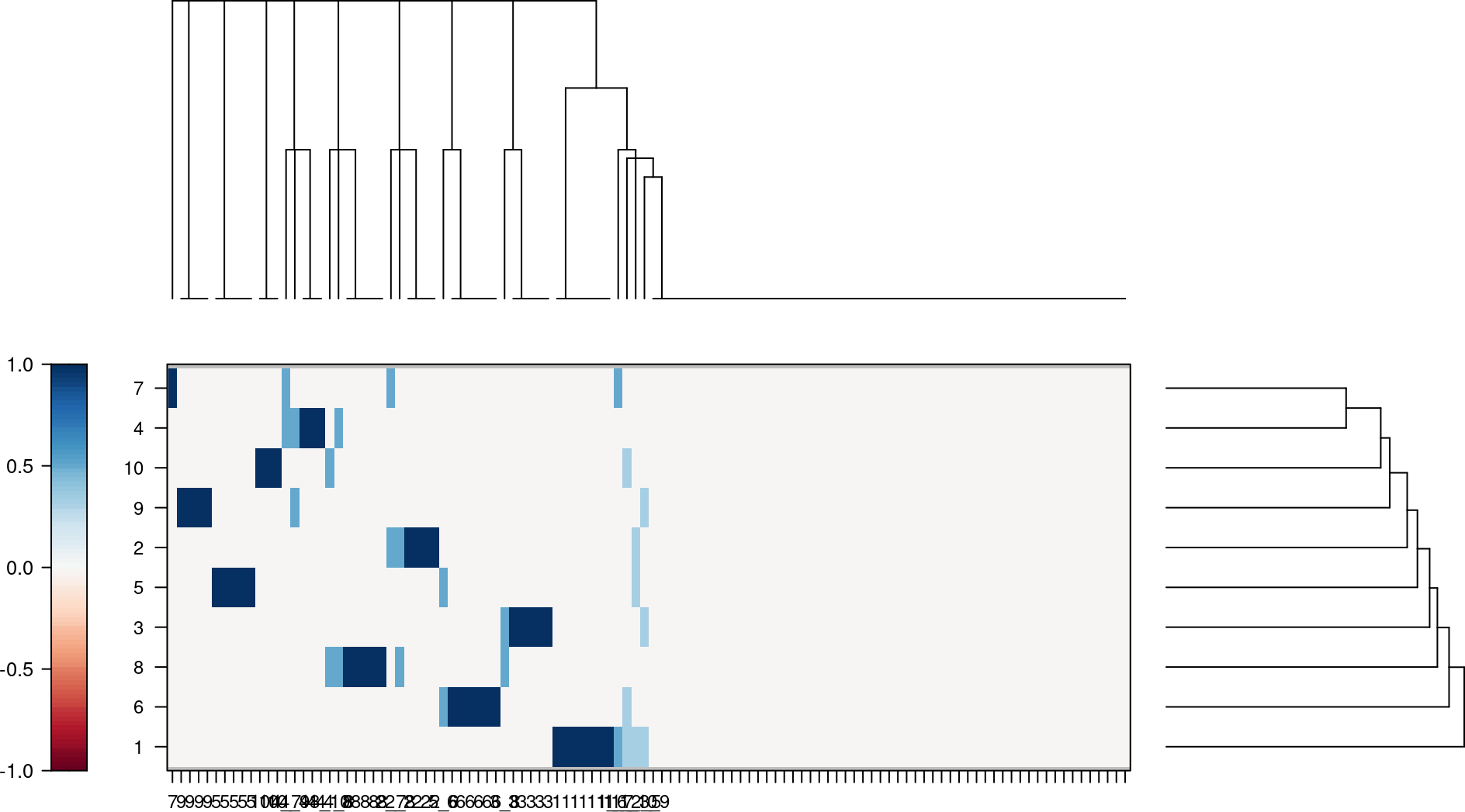Figure 2: Heatmap of the probabilsitic perturbation matrix colnames(data) <- sample(seq_len(Pgenes), ncol(data), replace = TRUE) res <- nempi(data, Gamma = Gamma) fit <- pifit(res, simmini, data) print(fit$auc)
##  0.7788576

## 3.4 Final perturbation matrix

The final perturbation matrix $$\Omega$$ over all samples is slightly different from the matrix $$\Gamma$$ we used as input. $$\Gamma$$ only denotes the most upstream P-gene perturbed. E.g., if A is upstream of B, than $$\Gamma$$ only denotes a perturbation of A, even though B is also perturbed in every samples in which B is perturbed. We can compute it by the matrix multiplication $$\Omega = \phi^T \times \Gamma$$.

Omega <- t(mnem::transitive.closure(res$res$adj)) %*% res\$Gamma
epiNEM::HeatmapOP(Omega, col = "RdBu", cexRow = 0.75, cexCol = 0.75, bordercol = "transparent",
xrot = 0, dendrogram = "both")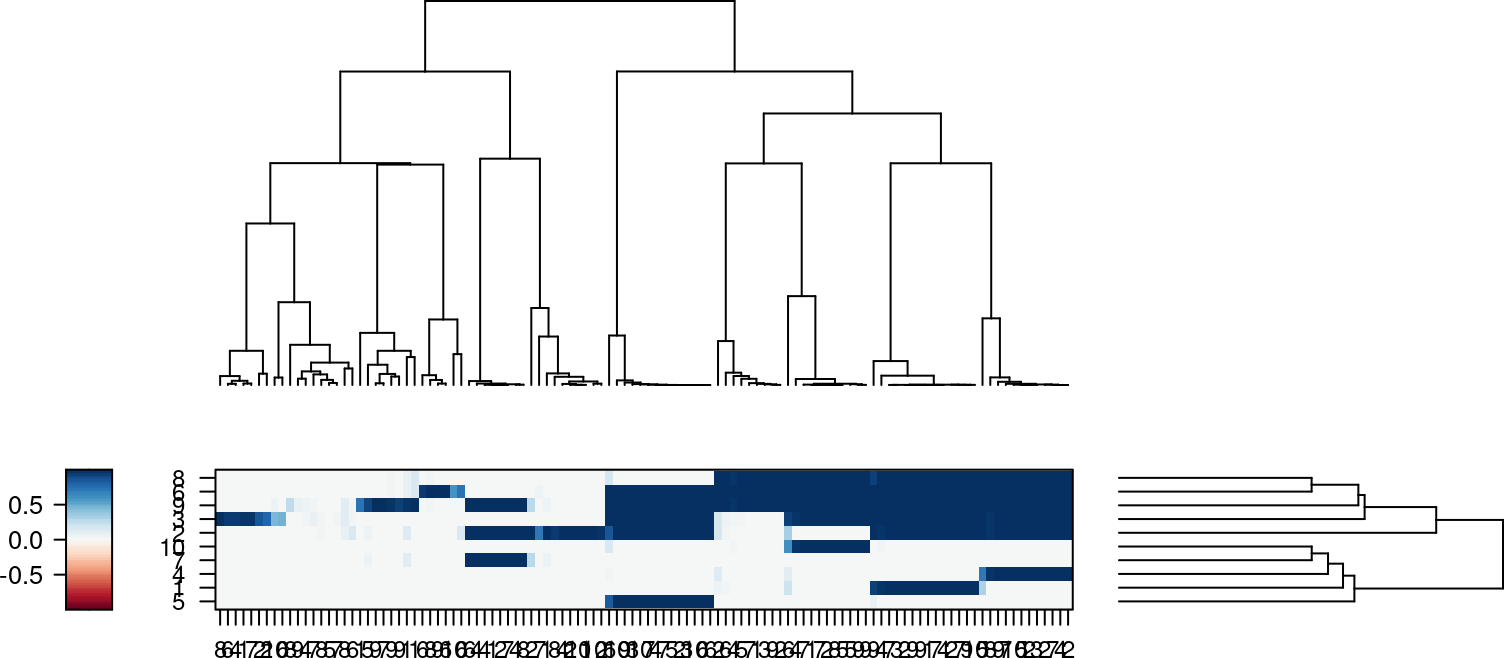# 4 Session information

sessionInfo()
## R version 4.2.0 RC (2022-04-21 r82226)
## Platform: x86_64-pc-linux-gnu (64-bit)
## Running under: Ubuntu 20.04.4 LTS
##
## Matrix products: default
## BLAS:   /home/biocbuild/bbs-3.16-bioc/R/lib/libRblas.so
## LAPACK: /home/biocbuild/bbs-3.16-bioc/R/lib/libRlapack.so
##
## locale:
##   LC_CTYPE=en_US.UTF-8       LC_NUMERIC=C
##   LC_TIME=en_GB              LC_COLLATE=C
##   LC_MONETARY=en_US.UTF-8    LC_MESSAGES=en_US.UTF-8
##   LC_PAPER=en_US.UTF-8       LC_NAME=C
##  LC_MEASUREMENT=en_US.UTF-8 LC_IDENTIFICATION=C
##
## attached base packages:
##  stats     graphics  grDevices utils     datasets  methods   base
##
## other attached packages:
##  nempi_1.5.0      mnem_1.13.0      BiocStyle_2.25.0
##
## loaded via a namespace (and not attached):
##    amap_0.8-18              Rtsne_0.16               colorspace_2.0-3
##    RcppEigen_0.3.3.9.2      ellipsis_0.3.2           class_7.3-20
##    modeltools_0.2-23        mclust_5.4.9             Linnorm_2.21.0
##   clue_0.3-60              proxy_0.4-26             flexmix_2.3-17
##   fansi_1.0.3              splines_4.2.0            robustbase_0.95-0
##   knitr_1.38               wesanderson_0.3.6        jsonlite_1.8.0
##   apcluster_1.4.9          cluster_2.1.3            kernlab_0.9-30
##   sfsmisc_1.1-13           png_0.1-7                latex2exp_0.9.4
##   snowfall_1.84-6.1        graph_1.75.0             BiocManager_1.30.17
##   compiler_4.2.0           assertthat_0.2.1         Matrix_1.4-1
##   fastmap_1.1.0            limma_3.53.0             cli_3.3.0
##   formatR_1.12             htmltools_0.5.2          tools_4.2.0
##   BoolNet_2.1.5            igraph_1.3.1             gtable_0.3.0
##   glue_1.6.2               epiNEM_1.21.0            dplyr_1.0.8
##   gmodels_2.18.1           Rcpp_1.0.8.3             jquerylib_0.1.4
##   vctrs_0.4.1              gdata_2.18.0             nlme_3.1-157
##   fpc_2.2-9                xfun_0.30                fastcluster_1.2.3
##   stringr_1.4.0            ggm_2.5                  lifecycle_1.0.1
##   gtools_3.9.2             XML_3.99-0.9             statmod_1.4.36
##   DEoptimR_1.0-11          MASS_7.3-57              zoo_1.8-10
##   scales_1.2.0             minet_3.55.0             parallel_4.2.0
##   RBGL_1.73.0              RColorBrewer_1.1-3       yaml_2.3.5
##   ggplot2_3.3.5            sass_0.4.1               bdsmatrix_1.3-4
##   naturalsort_0.1.3        fastICA_1.2-3            latticeExtra_0.6-29
##   stringi_1.7.6            highr_0.9                randomForest_4.7-1
##   e1071_1.7-9              permute_0.9-7            BiocGenerics_0.43.0
##   pcalg_2.7-6              rlang_1.0.2              pkgconfig_2.0.3
##   prabclus_2.3-2           matrixStats_0.62.0       evaluate_0.15
##   lattice_0.20-45          purrr_0.3.4              tidyselect_1.1.2
##   magrittr_2.0.3           bookdown_0.26            R6_2.5.1
##   magick_2.7.3             generics_0.1.2           DBI_1.1.2
##   pillar_1.7.0             mgcv_1.8-40              abind_1.4-5
##  nnet_7.3-17              tibble_3.1.6             tsne_0.1-3.1
##  crayon_1.5.1             utf8_1.2.2               ellipse_0.4.2
##  rmarkdown_2.14           jpeg_0.1-9               grid_4.2.0
##  flexclust_1.4-1          data.table_1.14.2        vegan_2.6-2
##  Rgraphviz_2.41.0         digest_0.6.29            diptest_0.76-0
##  stats4_4.2.0             munsell_0.5.0            bslib_0.3.1